# 10th Physics | Light | Some Important Terms Related to Spherical Mirrors

## Some Important Terms Related to Spherical Mirrors

The important terms related to spherical mirrors are –

### CENTRE OF CURVATURE (C)

The centre of curvature of a spherical mirror is the centre of the imaginary hollow sphere of glass, of which the spherical mirror is a part. The centre of curvature is usually denoted by the letter C. The centre of curvature of a concave mirror is in front of it and the centre of curvature of convex mirror is behind it as shown in the figure 1.

### RADIUS OF CURVATURE (R)

The radius of curvature of a spherical mirror is the radius of the imaginary hollow sphere of glass, of which the spherical mirror is a part. The radius of curvature is usually denoted by the letter ‘R’.

In figure 1 (a) the distance PC is the radius of curvature of a concave mirror and in figure (b) the distance PC is the radius of curvature of a convex mirror.

### POLE (P)

The centre of the reflecting surface of a spherical mirror is called its pole. It is usually denoted by the letter ‘P’ in each concave and convex mirror. The pole of a spherical mirror lies on the surface of the mirror as shown in figure 1 (a, b).

### PRINCIPAL AXIS

The principal axis of a spherical mirror is the straight line passing through the centre of curvature C and pole P of a spherical mirror, produced on both sides.

In the figure 1 (a, b) x x¢ is the principal axis of a concave and convex mirror.

### APERTURE

The portion of a mirror from which the reflection of light actually takes place is called the aperture of the mirror. It is also called linear aperture of the mirror. The aperture of a spherical mirror is denoted by the diameter of its reflecting surface. In the figure 1 (a, b) MN represent the aperture of concave and convex mirror.

Note:      In fact the aperture of a mirror represents the size of the mirror.Figure 1

### Principal Focus Of A Concave Mirror

The principal focus of a concave mirror is a point on the principal axis of the mirror, at which all the incident rays parallel to the principal axis, and close to it, actually meet (converge) after reflection from the mirror.

A concave mirror always have a real focus. It always lies in front of the concave mirror and is denoted by the letter ‘F’. as shown in the figure 2.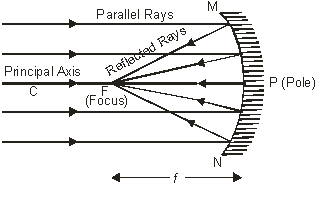Figure 2

### FOCAL LENGTH OF A CONCAVE MIRROR

Focal length of a concave mirror is the distance between the principal focus ‘F’ and the pole ‘P’ of the mirror. It is represented by the letter ‘f’. In the figure 2, PF = f = focal length of the concave mirror.

### PRINCIPAL FOCUS OF A CONVEX MIRROR

The principal focus of a convex mirror is a point on the principal axis of the mirror, at which all the incident rays parallel to the principal axis and close to it, appears to diverge, after reflection from the mirror.

A convex mirror has a virtual focus. It always lies behind the convex mirror and is denoted by the letter ‘F’ as shown in the figure 3.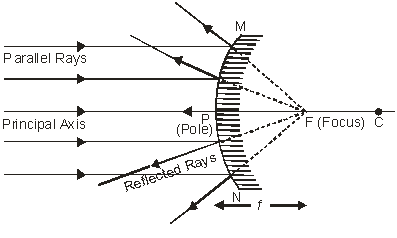Figure 3

### FOCAL LENGTH OF A CONVEX MIRROR

Focal length of a convex mirror is the distance between the principal focus (F) and the pole ‘P’ of the mirror. It is represented by the letter ‘f ‘

In the figure 3, PF = f = focal length of the convex mirror.

## RELATION BETWEEN RADIUS OF CURVATURE AND FOCAL LENGTH OF A SPHERICAL MIRROR

For spherical mirror having small aperture, the principal focus ‘F’ lies exactly at the midpoint of the pole P and the centre of curvature C as shown in the figure 12 & 13. Therefore the focal length of a spherical mirror (concave or convex) is equal to half of its radius of curvature.$\displaystyle f=\frac{R}{2}\ \ \Rightarrow \ \ R=2\ f$

### RULES FOR TRACING IMAGES FORMED BY CONCAVE MIRRORS

When an object is placed in front of a concave mirror, its image is formed by reflection in the mirror. To trace this image the four rays of light are commonly used, out of which we take any two rays of light (starting from the object) whose paths, after reflection from the mirror, are known to us and easy to draw. We may call them (the four rays) as rules for tracing images in concave mirrors. These are as follows :

(i) The incident ray of light which is parallel to the principal axis of a concave mirror, actually passes through the principal focus of the mirror after reflection from the mirror as shown in the figure 4.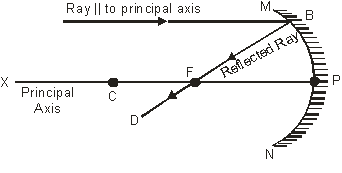Figure 4

(ii) The incident ray of light passing through the centre of curvature at a concave mirror is reflected back along the same path i.e. such a ray follows the same path after the reflection, but in the opposite direction as shown in the figure 5.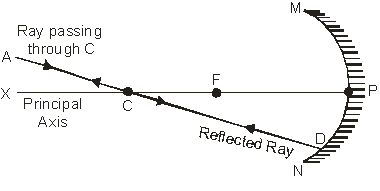Figure 5

(iii) Incident ray of light passing through the focus of a concave mirror becomes parallel to the principal axis of the mirror after reflection as shown in the figure 6.Figure 6

(iv) Incident ray of light which is incident (obliquely) at the pole P of the concave mirror is reflected back making the same angle with the principal axis of the concave mirror as shown in the figure 7.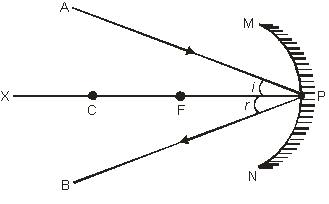Figure 7

## FORMATION OF DIFFERENT TYPES OF IMAGES BY A CONCAVE MIRROR IN DIFFERENT POSITIONS OF THE OBJECT

The type of image formed by a concave mirror primarily depends on the position of the object in front of the mirror. When an object is moved closer to the mirror, starting from infinity, the following six cases arise :

### (i) When the object is at infinity

When the object is at infinity, the rays of light starting from the object on travelling such a large distance are assumed to become parallel to each other while falling on the mirror. These parallel rays of light may fall on the mirror in the following two ways.

All the rays become parallel to the principal axis of the mirror as shown in the figure 8.

All the rays falling obliquely to the principal axis as shown in the figure 9.Figure 8Figure 9

In the above two cases image is formed at the focus of the mirror.     Therefore we can say that when the object is at infinity. The image is

(a) formed at the focus ‘F’ of the mirror.

(b) real and inverted

(c) highly diminished, point sized.

### (ii) When the object is beyond the centre of curvature ‘C’ of concave mirror

When the object is placed beyond the centre of curvature ‘C’ of the concave mirror (figure 10). The image is

(a) formed between the Focus ‘F’ and the centre of curvature ‘C’ of the concave mirror.

(b) real and inverted

(c) smaller in size i.e. diminished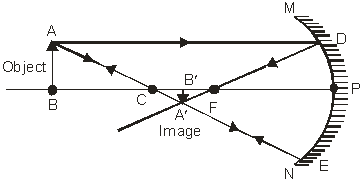Figure 10

### (iii) When the object is at the centre of curvature of concave mirror

When the object is placed at the centre of curvature of concave mirror (figure 11). The image is

(a) formed at the centre of curvature ‘C’ of the concave mirror.

(b) real and inverted.

(c) of the same size as that of the object.Figure 11

### (iv) When the object is placed between the centre of curvature ‘C’ and Focus ‘F’ of concave mirror

When the object is placed between the centre of curvature ‘C’ and Focus ‘F’ of concave mirror as shown in figure 12. The image is

(a) formed beyond centre of curvature ‘C’ of concave mirror

(b) real and inverted.

(c) enlarged, i.e. Larger in size than the object.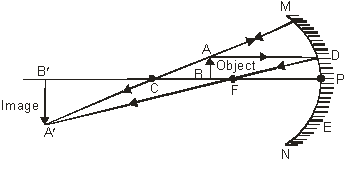Figure 12

### (v) When the object is at the Focus ‘F’ of a concave mirror

When the object is placed at the focus ‘F’ of a concave mirror as shown in the figure 13. The image is

(a) formed at infinity.

(b) real and inverted.

(c) highly enlarged, magnified.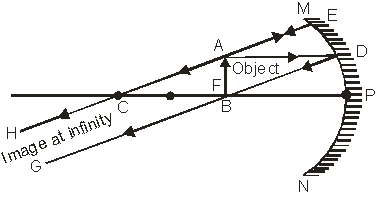Figure 13

### (vi) When the object is placed between Focus ‘F’ and pole ‘P’ of the concave mirror

When the object is placed between the Focus ‘F’ and Pole ‘P’ of the concave mirror as shown in the figure 14. The image is

(a) formed behind the mirror.

(b) virtual and erect.

(c) enlarged, larger than the size of the object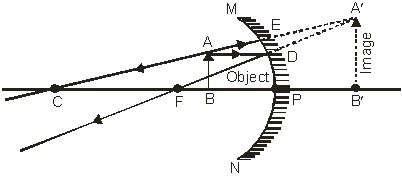Figure 14

Image formation by a concave mirror for different positions of the object:

 Position of the Object Position of the image Size of the image Nature of the image At infinity At the focus F Highly diminished, point-sized Real and inverted Beyond C Between F and C Diminished Real and inverted At C At C Same size Real and inverted Between C and F Beyond C Enlarged Real and inverted At F At infinity Highly enlarged Real and inverted Between P and F Behind the mirror Enlarged Virtual and erect

## USES OF CONCAVE MIRRORS

Some of the practical uses of concave mirrors are :

(i) Concave mirrors are used as reflectors in torches, search lights and head lights of motor vehicles etc. to get powerful and parallel beams of light.

(ii) Concave mirrors are used as shaving mirrors to see a larger image of the face.

(iii) Concave mirrors are used by dentists to see the larger images of the teeth of patients.

(iv) Large concave mirrors are used to concentrate sunlight to produce heat in solar furnaces.

## METHOD TO FIND OUT THE APPROXIMATE FOCAL LENGTH OF A CONCAVE MIRROR

To find out the approximate focal length of a concave mirror, focus a distant object (at infinity) on a screen by using a concave mirror whose focal length is to be determined. The sharp image of this object will be formed at the focus of the concave mirror. The distance of the image so formed from the concave mirror is equal to the focal length of concave mirror. Measure this distance with the help of a scale. It will give us the approximate focal length of the concave mirror.

## RULES FOR TRACING IMAGES FORMED BY CONVEX MIRRORS

When an object is placed in front of a convex mirror, its image is formed by reflection in the mirror. To trace this image the four rays of light are commonly used, out of which we take any two rays of light (starting from the object) whose paths, after reflection from the mirror, are known to us and easy to draw. We may call them (the four rays) as rules for tracing images in convex mirrors. These are as follows :

(i) The incident ray of light parallel to the principal axis of a convex mirror, appears to be coming from its focus ‘F’ after reflection from the mirror as shown in the figure 15.

In the figure the incident ray AB which is parallel to the principal axis, after reflection from the convex mirror follows the path BD. This reflected ray BD appears to come from the Focus ‘F’ behind the convex mirror.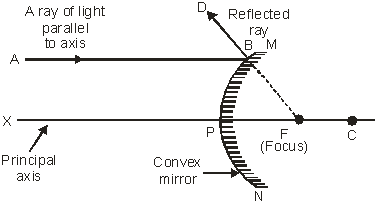Figure 15

(ii) The incident ray of light which appears to pass through the centre of curvature ‘C’ of a convex mirror is reflected back along the same path, as shown in the figure 16.

In the figure the incident ray AD appears to pass through the centre of curvature ‘C’ behind the convex mirror, after reflection follows the same path DA.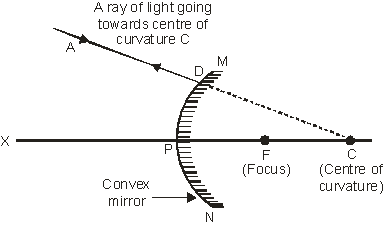Figure 16

(iii) The incident ray of light which appears to pass through the focus ‘F’ of a convex mirror becomes parallel to the principal axis after reflection as shown in the figure 17.

In the figure the incident ray AE appears to pass through the Focus ‘F’ behind the mirror, after reflection follows the path EG. This reflected ray EG is parallel to the principal axis of the convex mirror.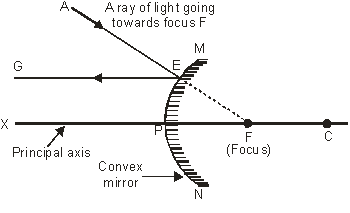Figure 17

(iv) The incident ray of light which is falling obliquely at some angle towards the pole ‘p’ of a convex mirror is reflected back making the same angle with the principal axis, as shown in the figure 18.

In the figure the incident ray AP which is falling on the pole ‘P’ of the convex mirror making some angle of incidence with the normal, after reflection follows the path PH, thereby making an angle of reflection ‘r’ with the normal such that the angle of incidence ‘i’ is equal to the angle of reflection r i.e. Ð i = Ðr.Figure 18

## FORMATION OF DIFFERENT TYPES OF IMAGES BY A CONVEX MIRROR IN DIFFERENT POSITIONS OF THE OBJECT

The type of image formed by a convex mirror primarily depends upon the position of the object in front of the mirror. When an object is moved closer to the mirror, starting from infinity, the following two cases arise :

(i) When the object is at infinity

When the object is at infinity, the rays of light starting from the object on travelling such a large distance are assumed to become parallel to each other while falling on the mirror. These parallel rays of light may fall on the mirror in the following two ways–

(a) All the incident rays become parallel to the principal axis of the convex mirror as shown in the figure 19.

(b) All the incident rays falling obliquely to the principal axis, as shown in the figure 20.Figure 19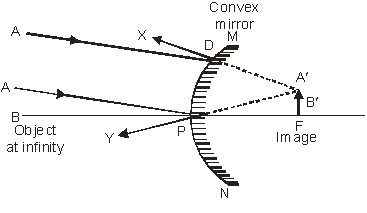Figure 20

In the above two cases image is formed at the Focus ‘F’ of the convex mirror, behind the mirror.

Therefore we can say that when the object is at infinity, the image is formed

(a) At the Focus ‘F’ behind the mirror.

(b) Virtual and erect.

(c) Highly diminished, point sized (much smaller than the object).

(ii) When the object is at finite distance from the convex mirror. i.e. between infinity and the pole P of the convex mirror :.

When the object is at finite distance i.e. anywhere between infinity and the pole ‘P’ of the convex mirror (figure 21). The image is formed

(a) Between the pole ‘P’ and Focus ‘F’ behind the convex mirror.

(b) Virtual and erect.

(c) Diminished, smaller than the objectFigure 21

Image formation by a convex mirror for different positions of the object:

 Position of the Object Position of the image Size of the image Nature of the image At infinity At the focus F, behind the mirror Highly diminished, point-sized Virtual and erect Between infinity and the pole P of the mirror Between P and F, behind the mirror Diminished Virtual and erect

## USES OF CONVEX MIRROR

Some of the practical uses of convex mirrors are :

(i) A convex mirror is used as a reflector in street lamps. As a result, light from the lamp diverges over a large area.

(ii) Convex mirrors are used as rear-view mirrors in automobiles (like cars, trucks and buses) to see the objects (traffic) at the rear side.

The convex mirror is preferred as a rear view mirror because

(a) A convex mirror always produces an erect image of the objects.

(b) The size of the image formed by a convex mirror is highly diminished or much smaller than the object, due to which it covers a wide field of view, which enables the driver to view much larger area of the traffic behind him than would be possible with a plane mirror.

## METHOD TO DISTINGUISH BETWEEN A PLANE MIRROR, A CONCAVE MIRROR AND A CONVEX MIRROR WITHOUT TOUCHING THEM

To distinguish between a plane mirror, a concave mirror and a convex mirror, without touching them, we simply look at the image of our face in the three mirrors, turn by turn.

All of them will produce an image of our face but of different types.

A plane mirror will produce virtual and erect image of the same size as our face and we will look our normal self.

A concave mirror will produce a virtual, erect and magnified image of our face i.e. our face will look much bigger.

A convex mirror will produce a virtual, erect but diminished image of our face i.e. our face will look much smaller.

This site uses Akismet to reduce spam. Learn how your comment data is processed.